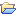# Frequencies

Contains nodes that compute several kinds of frequencies

This category contains 8 nodes.

##### DF

This node computes the number of documents that contain the terms of the input bag of words table.

##### Frequency Filter

Filters terms with a certain frequency value.

##### ICF

Computes the inverse category frequency (icf) of each term according to the given set of documents, categories of documents respectively, and adds a […]

##### IDF

Computes the inverse document frequency (idf) of each term according to the given set of documents and adds a column containing the idf value.

##### NGram Creator

Creates ngrams of documents.

##### Term Co-Occurrence Counter

Counts the number of co-occurrences for the given list of terms within the different parts e.g. sentence, paragraph, section and title of […]

##### Term Document Entropy

This node computes the informational entropy of each term in each document. If a term occurs once in every document, its entropy for each document […]

##### TF

Computes the relative term frequency (tf) of each term according to each document and adds a column containing the tf value.